# NCERT Solutions Class 10 Maths Chapter 6 – Download PDF

Get here NCERT Solutions Class 10 Maths Chapter 6. These NCERT Solutions for Class 10 of Maths subject includes detailed answers of all the questions in Chapter 6 – Triangles provided in NCERT Book which is prescribed for class 10 in schools.

Resource: National Council of Educational Research and Training (NCERT) Solutions
Class: 10th Class
Subject: Maths
Chapter: Chapter 6 – Triangles

## NCERT Solutions Class 10 Maths Chapter 6 – Triangles

NCERT solutions for class 10 maths chapter 6 triangles is provided here for free PDF download to help students in the preparation for their board exam in a better way. It is one of the best tools to prepare chapter 6 Triangles for maths exam. Students are suggested to practice these NCERT Solutions on a regular basis to excel in the 10th standard examination.

Triangle is a scoring chapter for 10th class, where derivations and problems with solutions are to be practiced by students to achieve expertise. The important topics covered in this chapter 6 are:

• Introduction to triangle
• Similar figures
• Similarity of triangles
• Areas of similar triangles
• Pythagoras theorem

### NCERT Solutions For Class 10 Maths Chapter 6 – Triangles – Exercise 6.1

Question 1:

Fill in the blanks

A: All circles are ________ (Similar, Congruent)

Solution: All circles are similar.

B: All squares are ________ (Congruent, similar)

Solution: All squares are similar.

C: All _________ triangles are similar. (Isosceles, equilateral)

Solution: All equilateral triangles are similar.

D: Two polygons having the same number of sides are similar, if

(i) Their corresponding angles are _______ and

(ii) Their corresponding sides are __________. (Proportional, equal)

Solution: Two polygons having the same number of sides are similar, if (i) their corresponding angles are equaland (ii) their corresponding sides are proportional

Question 2:

Give examples for the following, two of each.

A: Similar figures

B: Non-similar figures

Solution:

A: Two ten dollar notes and two ten cent coins.

B: One 5 cent coin and 10 cent coin and one 5 dollar note and ten dollar note.

Question 3:

Find out whether the quadrilaterals that are given below are similar.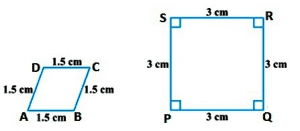Solution:

Since, the corresponding angles of both the figures are not equal, the figures given are not similar.

### NCERT Solutions For Class 10 Maths Chapter 6 – Triangles – Exercise 6.2

Question 1:

From the given figures , we get that ST ǁ QR. Find TR in (i) and PS in (ii).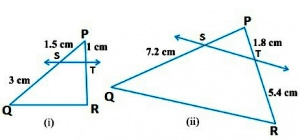Solution:

In the triangle, PQR, ST ǁ QR (given)

Therefore, PS/SQ = PT/TR [Using the basic Proportionality theorem]

=> 1.5/3 = 1/ TR

=> ∑ TR = 3/1.5

TR = 3 x 10/15 = 2cm

Hence, TR = 2cm

(ii) In triangle PQR, ST ǁ QR (given)

Therefore, PS/ SQ = PT / TR [Using the basic proportionality theorem]

= > PS/7.2 = 1.8/5.4

= > PS = 1.8 x 7.2/5.4 = 18/10 x 72/10 x 10/54 = 24/10

= > PS = 2.4

Hence, PS = 2.4 cm

Question 2:

A and B are two points on the sides of XY and XZ respectively of a XYZ triangle. State whether AB ǁ YZ for the following given cases:

1: XA = 3.9 cm, AY = 3 cm XB = 3.6 cm and BZ= 2.4 cm

2: XA = 4 cm, YA = 4.5 cm, XB = 8 cm and ZB = 9 cm

3: XY = 1.28 cm, XZ = 2.56 cm, XA = 0.18 cm and XB = 0.63 cm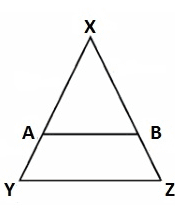Solution:

In triangle XYZ, A and B are the two points on sides XY and XZ respectively.

1:  XA = 3.9 cm and AY = 3 cm (given)

XB = 3.6 cm, BZ = 2.4 cm (Given)

Therefore, XA/AY = 3.9/3 = 39/30 = 13/10 = 1.3 [Using the basic proportionality theorem]

And, XB/BZ = 3.6/2.4 = 36/24 = 3/2 = 1.5

So, XA/AY ≠ XB/BZ

Hence, AB is not parallel to YZ.

2: XA = 4cm, YA = 4.5 cm, XB = 8cm, ZB= 9cm

Therefore, XA/YA = 4/4.5 = 40/45 = 8/9 [Using the basic proportionality theorem]

And, XB/ZB = 8/9

So, XA/YA = XB/ZB

Hence, AB is parallel to YZ

3: XZ = 1.28 cm, XZ = 2.56 cm, XA= 0.18 cm, XB= 0.36 cm (Given)

Here, AY = XY – XA = 1.28-0.18 = 1.10 cm

And, BZ = XZ – XB = 2.56 – 0.36 = 2.20 cm

So, XA/AY = 0.18/1.10 = 18/110 = 9/55 – – – – – – – – – – – – (1)

And, XA/BZ = 0.36/2.20 = 36/220 = 9/55 – – – – – – – – – – – (2)

Therefore, XA/AY = XB/BZ

Hence, AB is parallel to XY.

Question 3:

From the given figure, we see that AB ǁ RQ and AC ǁ RS, prove that PB/BQ = PC/PS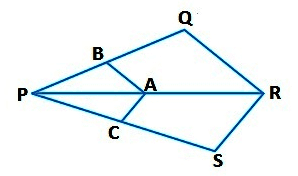Solution:

From the given figure, we get AB ǁ RQ

By using the basic proportionality theorem, we get,

PB/BQ = PA/ PR – – – – – – – – (1)

Similarly, AC ǁ RS

Therefore, PC/PS = PA/PR – – – – (2)

From (1) and (2) we get,

PB/BQ = PC/PS

Question 4:

From the figure we get, ST ǁ PR and SU ǁ PT. Prove that QU/UT = QT/TR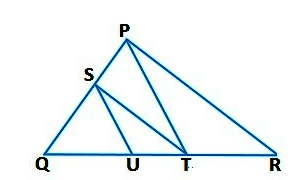Solution:

In triangle PQR, ST ǁ PR (Given)

Therefore, QS/SP= QT/TR – – – – – – – (1) [Using the proportionality Theorem]

In triangle PQR, SU ǁ PT (Given)

Therefore, QS/SP = QU/UT – – – – – – (2) [Using the basic proportionality theorem]

From equation (1) and (2) we get,

QT/TR = QU/UT

Question 5:

From the following figure we get, XZ ǁ AC and XZ ǁ AD, show that YZ ǁ CD.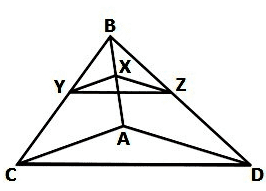Solution:

In triangle BCA, XY ǁ AC (Given)

Therefore, BX/XA = BY/YC – – – – – – – (1) [Using the basic proportionality theorem]

In triangle BCA, XY ǁ AC (Given)

Therefore, BX/XA = BZ/ZD – – – – – – – (2) [Using the basic proportionality theorem]

From the equation (1) and (2) we get,

BY/YC = BZ/ZD

In triangle BCA, YZ ǁ CD [By converse of the basic proportionality theorem]

Question 6:

From the figure, three points X, Y and Z are points on AB, AC and AD respectively such that XY ǁ BC and XZ ǁ BD. Show that YZ ǁ CD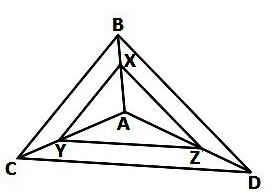Solution:

In triangle ABC, XY ǁ BC (Given)

Therefore, AX/XB = AY/YC – – – – – – – (1) [Using the basic proportionality theorem]

In triangle ABC, XZ ǁ BD (Given)

Therefore, AX/XB = AZ/ ZD – – – – – – – (2) [Using the basic proportionality theorem]

From the equations (1) and (2), we get

AY/ YC = AZ/ZD

In triangle ACD, YZ ǁ CD [By the converse of basic proportionality theorem]

Question 7:

Using the basic proportionality theorem, prove that a line drawn through the midpoints of one side of a triangle is parallel to the other side that bisects the third side.

Solution: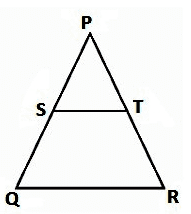From the given diagram we get,

The triangle PQR in which S is the midpoint of P and Q such that PS=SQ

A line parallel to QR intersects PR at T such that ST ǁ QR

To prove: T is the midpoint of PR

Proof: S is the midpoint of PQ

Therefore, PS=SQ

=>PS/QS = 1 – – – – – – – (1)

In triangle PQR, ST ǁ QR,

Therefore, PS/SQ = PT / TR [Using the basic proportionality theorem]

=>1 = PT/ TR [from equation (1)]

Therefore, PT = TR

Hence, T is the midpoint of PR

Question 8:

Prove that the line joining the midpoints of any two sides of a triangle is parallel to the third side by using the converse of basic proportionality theorem.

Solution: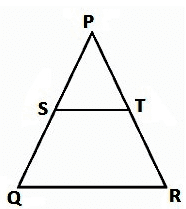Given:

From the triangle PQR in which ST are the midpoints of PQ and PR respectively such that PS = SQ and PT= TR

To prove: ST ǁ QR

Proof: S is the midpoint of PQ (Given)

Therefore, PS = SQ

=>PS/QS = 1 – – – – – – – – – (1)

Also, T is the midpoint of PR (Given)

Therefore, PT= TR

=>PT/TR = 1 [from equation (1)]

From equation (1) and (2) we get,

PS/ QS = PT/ TR

Hence, ST ǁ QR [By the converse of the basic proportionality theorem]

Question 9:

PQRS is a trapezium in which PQ ǁ SR and its diagonals intersect each other at a point A. show that PA/QA = RA/SA

Solution: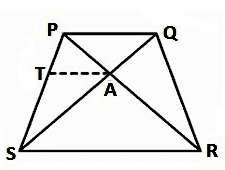Given:

PQRS is a trapezium in which PQ ǁ RS in which the diagonals PR and QS intersect each other at A.

To prove: PA/QA = RA/ SA

Construction: Through A, draw TA ǁ SR ǁ PQ

Proof: In triangle PSR, we have

AT ǁ SR (By construction)

Therefore, PT/TS = PA/RA – – – – – – – (1) [Using the basic proportionality theorem]

In triangle PQS, we have

AT ǁ PQ (by construction)

Therefore, ST/TP = SA/QA – – – – – – – – – (2)  [Using the basic proportionality theorem]

From the equations (1) and (2) we get,

PA/RA = QA / SA

=>PA / QA = RA/SA

Question 10:

The diagonals of a quadrilateral PQRS intersect each other at the point A such that PA/QA = RA/SA. Show that PQRS is a trapezium.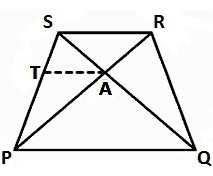Solution:

Given:

Quadrilateral PQRS in which diagonals PR and QS intersect each other at A such that PA/ QA = RA/SA

To prove: PQRS is a trapezium

Construction: Through A, draw line TA, where TA ǁ PQ, which meets PS at T

Proof: In triangle SPQ, we have

TA ǁ PQ

Therefore, ST/TP = SA/AQ – – – – – – (1) [Using the basic proportionality theorem]

Also, PA/QA = RA/SA (Given)

=>PA/ RA = QA/ SA

=> RA/PA = QA/SA

=> SA/AQ = RA/PA – – – – – – – – (2)

From the equations (1) and (2) we get,

ST / TP = RA/ PA

Therefore, By using converse of Basic proportionality theorem,

TA ǁ SR also TA ǁ PQ

=>PQ ǁ SR

Hence, quadrilateral PQRS is a trapezium with PQ ǁ RS

### NCERT Solutions For Class 10 Maths Chapter 6 – Triangles – Exercise 6.3

Question 1:

Which of the following triangle pairs are similar? State the similarity criterion you used to determine the similarity of the triangles.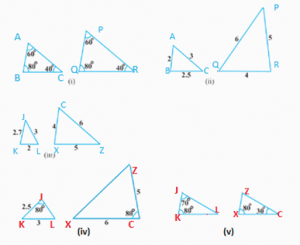Solution:

(i)For  ΔABC and ΔPQR:

∠A=∠P = 60(Given)

∠B =∠Q = 80o(Given)

∠C =∠R = 40o(given)

∴ Δ ABC ~ ΔPQR (AAA similarity criteria)

(ii)For ΔJKL and ΔZXC

JK/XC = KL/CZ = JL/XZ
∴  ΔJKL~ ΔZXC (SSS similarity criterion)

(iii) For ΔJKL and ΔZXC:
JK = 2.7, KL = 2, LJ = 3, ZX = 5, XC = 4, CZ = 6
KL/ZX = 2/4 = 1/2
JL/ZC = 3/6 = 1/2
JK/XC= 2.7/5 = 27/50
Here, KL/ZX = LJ/ZC ≠ JK/XC
Thus, ΔJKL and ΔZXC are not similar.

(iv) For ΔJKL and ΔZXC
JK = 2.5, KL = 3, ∠J = 80°, XC = 6, ZC = 5, ∠C = 80°
Here, JK/ZC = 2.5/5 = 1/2
And, KL/XC = 3/6 = 1/2
⇒ ∠K ≠ ∠C
Thus, ΔJKL and ΔZXC are not similar.

(v)  For ΔJKL, we have
∠J + ∠K + ∠L = 180° (sum of angles of a triangle)
⇒ 70° + 80° + ∠L = 180°
⇒ ∠L = 180° – 70° – 80°
⇒ ∠L = 30°
In ZXC, we have
∠Z + ∠X + ∠C = 180 (Sum of angles of Δ)
⇒ ∠Z + 80° + 30° = 180°
⇒ ∠Z = 180° – 80° -30°
⇒ ∠Z = 70°
In ΔJKL and ΔZXC, we have
∠J = ∠Z = 70°
∠K = ∠X = 80°
∠L = ∠C = 30°
Thus, ΔJKL ~ ΔZXC (AAA similarity criterion)

Question 2:

In the figure below, ΔJKL  ¼ ΔZXL, KJL=70O and ZLX = 70O. Find JLK, JKL AND LZX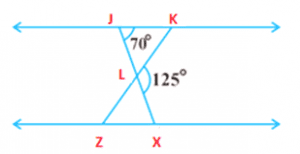Solution:

JLX is a straight line.
Thus, ∠JLK + ∠ KLX = 180°
⇒ ∠JLK = 180° – 125°
= 55°

In ΔJLK,
∠JKL+ ∠ KJL + ∠ JLK = 180°
(Sum of the measures of the angles of a triangle is 180º.)
⇒ ∠JKL + 70º + 55º = 180°
⇒ ∠JKL = 55°
Given that ΔLJK ~ ΔLXZ.

∴ ∠LZX = ∠LKJ  (Corresponding angles are equal in similar triangles)
⇒ ∠ LZX = 55°
∴ ∠LZX= ∠LKJ   (Corresponding angles are equal in similar triangles)

⇒ ∠LZX= 55°

Question 3:

Diagonals JX and KZ of a trapezium JKZX with JK || ZX intersect each other at the point L. With the help of similarity criterion for two triangles, prove that JL/LX = KL/KZ.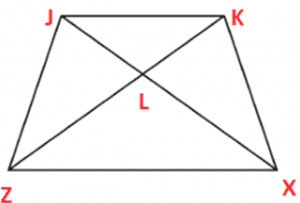Solution:

In ΔZLX and ΔJLK,
∠XZL = ∠JKL (Alternate interior angles as JK || XZ)
∠ZXL = ∠KJL (Alternate interior angles as JK || XZ)
∠ZLX = ∠KLJ (Vertically opposite angles)
∴ ΔZLX ~ ΔKLJ (AAA similarity criterion)

∴ ZL/KL = LX/LJ  (Corresponding sides are proportional)

⇒ LJ/LX = LK/LZ

Question 4:

In the given figure, LX/LZ = LJ/KX and 1 = 2. Prove that ΔKXZ ~ ΔJLX.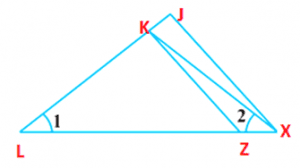Solution:

In ΔJLX, ∠KLX = ∠KXL
∴ KL = KX … (i)
Given, LX /LZ = LJ/KX
Using (i), we get
LX/LZ = LJ/LK ..(ii)

In ΔKLZ and ΔJLX,
LX/LZ = LJ/LK (using(ii))
∠L = ∠L
∴ ΔKZX ~ ΔJLX [SAS similarity criterion]

Question 5:

In the given figure, K and L are points on sides JZ and JX of ΔZXJ such that Z = JLK. Prove that ΔJZX ~ ΔJLK.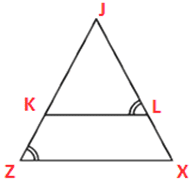Solution:

In ΔJZX and ΔJKL,
∠JLK = ∠XZK (Given)
∠J = ∠J (Common angle)
∴ ΔJZX ~ ΔJLK (By AA similarity criterion)

Question 6:

In the figure given, if ΔJZL  ΔJXK, prove that ΔJKL ~ ΔJZX.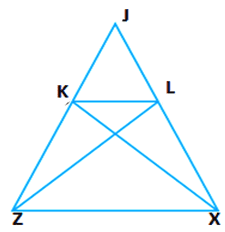Solution:

Given, that ΔJZL ≅ ΔJXK.
∴ JZ = JX [By cpct] … (i)
Also, JK = JL [By cpct] … (ii)
In ΔJKL and ΔJZX,

JK/JZ = JL/JX [Dividing equation (ii) by (i)]

∠J = ∠J [Common angle] ∴ ΔJKL ~ ΔJZX [By SAS similarity criterion]

Question 7:

In the figure given below, lines ZK and JX of ΔJZC intersect each other at the point L. Prove that:

(i) ΔZXL ~ ΔJKL
(ii) ΔZCK ~ ΔJCX
(iii) ΔZXL ~ ΔZKC
(iv) ΔLKJ ~ ΔCXJ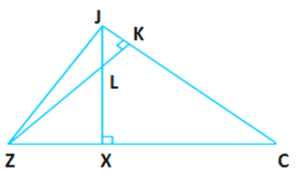Solution:

(i) In ΔZXL and ΔJKL,
∠ZXL = ∠JKL (Each 90°)
∠ZLX = ∠JLK (Vertically opposite angles)
Thus, by using AA similarity criterion,
ΔZXL ~ ΔJKL

(ii) In ΔZCK and ΔJCX,
∠ZKC = ∠JXC (Each 90°)

∠ZCK = ∠JCX (Common)
Hence, by using AA similarity criterion,
ΔZCK ~ ΔJCX
(iii) In ΔZXL and ΔZKC,
∠ZXL = ∠ZKC (Each 90°)

∠LZX = ∠KZC (Common)
Hence, by using AA similarity criterion,
ΔZXL ~ ΔZKC
(iv) In ΔLKJ and ΔCXJ,
∠LKJ = ∠CXJ (Each 90°)
∠LJK = ∠CJX (Common angle)
Hence, by using AA similarity criterion,
ΔLJK ~ ΔCXJ

Question 8:

In the given figure X is a point on the side JZ of a  parallelogram JKCZ and KX  intersect ZC at L. Prove that ΔJKX ~ ΔCLK.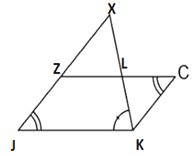Solution:

In ΔJKX and ΔCLX,
∠J = ∠C (Opposite angles of a parallelogram)
∠JXK = ∠CKL (Alternate interior angles as JX || KC)
∴ ΔJKX ~ ΔCLX (By AA similarity criterion)

Question 9:

In the given figure, JKC and AMP are two right triangles, right angled at B and M respectively, show that:

i) ΔJZC ~ ΔJKX

(ii) CJ/XJ = ZC/KX

Solution: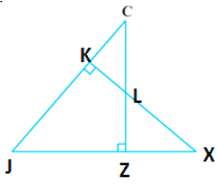(i) In ΔJZC and ΔJKX, we have

∠J= ∠J (common angle)

∠JZC = ∠JKX = 90° (each 90°)

∴ ΔJZC ~ ΔJKX (By AA similarity criterion)

(ii) Since, ΔABC ~ ΔAMP (By AA similarity criterion)

If two triangles are similar then the corresponding sides are equal,

Hence, CJ/XJ = ZC/KX

Question 10:

In the given figure CD and KH are the bisectors of ZCX and JKL respectively, such that D and H lie on sides ZX and JL of ΔZXC and ΔJKL respectively. If ΔZXC ~ ΔJKL. Prove that:

(i) CD/KH = ZC/JK
(ii) ΔCXD ~ ΔKLH
(iii) ΔDZC ~ ΔHKJ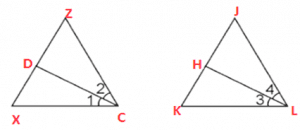Solution:

(i) Given that ΔZXC ~ ΔJKL
∴ ∠Z = ∠J, ∠X = ∠L, and ∠ZCX = ∠JKL
∠ZCX = ∠JKL
∴ ∠ZCD = ∠JGH (Angle bisector)
And, ∠DCX = ∠HKL (Angle bisector)
In ΔDZC and ΔJKH,
∠Z = ∠J (Proved above)
∠DCZ = ∠JKH (Proved above)
∴ ΔDZC ~ ΔJKH (By AA similarity criterion)

⇒ CD/KH = ZC/JK

(ii) In ΔXCD and ΔHKL,
∠XCD = ∠HK; (Proved above)
∠X = ∠L (Proved above)
∴ ΔXCD ~ ΔHKL (By AA similarity criterion)

(iii) In ΔZCD and ΔHJK
∠DCZ = ∠JKH (Proved above)
∠Z = ∠J (Proved above)
∴ ΔZCD ~ ΔHJK (By AA similarity criterion)

Question 11:

In the following figure, K is a point on side CL  of an isosceles triangle JCL, where JL= JC. If JZ  LC and KF CJ. Show that ΔJLZ ~ ΔFCK.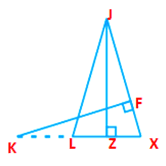Solution:

It is given that JLC is an isosceles triangle.
∴ JL = JC
⇒ ∠JLZ = ∠FCJ
In ΔJLZ and ΔFKC,
∠JDL = ∠CFK (Each 90°)
∠LJZ = ∠FKC (Proved above)
∴ ΔJLZ ~ ΔFCK (By using AA similarity criterion)

Question 12:

In the given figure sides ZX and XC and median ZV of a triangle ZXC are proportional to sides HJ and JL  and median HK of ΔPQR, respectively. Prove that ΔZCX ~ ΔHJL.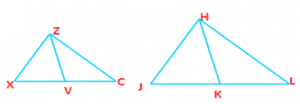Solution:

It is given that:

ΔZCX and ΔHJL, ZX, CX and median ZV of ΔZCX are proportional to sides HJ, JL and median HK of ΔHJL

Or, ZX/HJ = CX/JL= ZV/

To Prove: ΔZCX ~ ΔHJL

Proof: ZX/HJ = CX/JL = ZV/HK

⇒ ZX/HJ = CX/JL = ZV/HK (V is the mid-point of CX and K is the midpoint of JL)

⇒ ΔZXV ~ ΔHJK [SSS similarity criterion]

∴ ∠ZXV = ∠HJK [Corresponding angles of two similar triangles are equal]

⇒ ∠ZXC = ∠HJL

In ΔABC and ΔPQR

ZX/HJ = CX/JL ….(i)

∠CXZ = ∠HJL….. (ii)

Hence, from equation   (i) and (ii), we get

ΔCXZ ~ ΔHJL [By SAS similarity criterion]

Question 13:

J is a point on the side CK of a triangle CJK such that JLC = KJC. Prove that CJ2 = CK.LC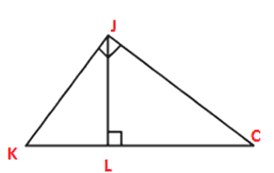Solution:

In ΔCJL and ΔJKC,
∠CLJ = ∠CJK (Given)
∠JCL = ∠KCJ (Common angle)
∴ ΔCJL ~ ΔJKC (By AA similarity criterion)
We know that corresponding sides of similar triangles are in proportion.

∴ CJ/KC =CL/JC

⇒ CJ2 = CK.LC.

Question 14:

In the given figure, sides ZX and ZC and median ZV of a triangle CXZ are proportional to sides JK and JH and median JL of another triangle JKH, respectively. Show that ΔCXZ~ ΔJKH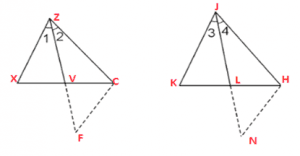Solution:

It is given that:

Two triangles ΔCXZ and ΔJKH in which ZV and JL are medians such that ZX/JK = CZ/JH = ZV/JL

To Prove: ΔZXC ~ ΔJKH

Construction: Produce ZV  to F such that ZV = VF. Connect CF. Similarly produce JL to N so that JL = LN, also connect HN.

Proof:

In ΔZXV and ΔVCF, we have

ZV = VF                                   [By Construction]

XV = VC                                   [∴ AP is the median]

And, ∠ZVX = ∠CVF                  [Vertically opp. angles]

∴ ΔZXV ≅ ΔCVF                       [By SAS criterion of congruence]

⇒ ZX = CF                                [CPCT] ….. (i)

Also, in ΔJKL and ΔLNH, we have

JL = LN                                     [By Construction]

KL = LH                                    [∴ PM is the median]

and, ∠JLK = ∠NLH                   [Vertically opposite angles]

∴ ΔJKL = ΔLHN                         [By SAS criterion of congruence]

⇒ JK = HN                               [CPCT]  …. (ii)

Now, ZX/JK = ZC/JH = ZV/JL

⇒ CF/HN = ZC/JH = ZV/JL … [From (i) and (ii)]

⇒ CF/HN = ZC/JH = 2ZV/2JL

⇒ CF/HN = ZC/JH = ZF/JN     [∴ 2AD = AE and 2PM = PN]

∴ ΔZCF ~ ΔJHN                        [By SSS similarity criterion]

Therefore, ∠2 = ∠4

Similarly, ∠1 = ∠3

∴ ∠1 + ∠2 = ∠3 + ∠4

⇒ ∠Z = ∠J … (iii)

Now, In ΔZXC and ΔJKH, we have

ZX/JK = ZC/JH       (Given)

∠Z = ∠J [From (iii)]

∴ ΔZXC ~ ΔJKH                             [By SAS similarity criterion]

Question 15:

A vertical pole of a length 6 m casts a shadow 4m long on the ground and at the same time, a tower casts a shadow 28 m long. Find the height of the tower.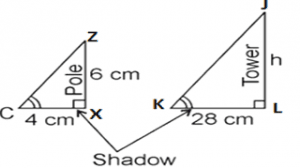Solution:

Length of the vertical pole = 6m (Given)

Shadow of the pole = 4 m (Given)

Let Height of tower = h m

Length of shadow of the tower = 28 m (Given)

In ΔZXC and ΔJKL,

∠C = ∠K (angular elevation of sum)

∠X = ∠L = 90°

∴ ΔZXC ~ ΔJKL (By AA similarity criterion)

∴ ZX/JL = XC/KL (when two triangles are similar corresponding sides are proportional)

∴ 6/h = 4/28

⇒ h = 6×28/4

⇒ h = 6 × 7

⇒ = 42 m

Therefore, the height of the tower is 42 m.

Question 16:

In the given figure if ZV and JM are medians of triangles ZXC and JKL, respectively. Where ΔZXC ~ ΔJKL prove that ZX/JK = ZV/JM.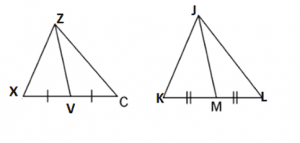Solution:

It is given that: ΔZXC ~ ΔJKL
We know that, the corresponding sides of similar triangles are in proportion

.∴ ZX/JK = ZC/JL = KC/KL … (i)
Also, ∠ Z= ∠J, ∠X = ∠K, ∠C = ∠L …(ii)
As ZV and JM are medians, their opposite sides will be divided by them

.∴ XV = XC/2 and KM = KL/2 …(iii)
From equations (i) and (iii), we have
ZX/JK = XV/KM …(iv)
In ΔZXV and ΔJKM,
∠X = ∠K [Using equation (ii)] ZX/JK = XV/KM [Using equation (iv)] ∴ ΔZXV ~ ΔJKM (By SAS similarity criterion)

⇒ ZX/JK = XV/KM = ZV/JL.

### NCERT Solutions For Class 10 Maths Chapter 6 – Triangles – Exercise 6.4

Question 1:

Let ∆ PQR ~ ∆ STU and their areas be, 64cm2 and 121cm2 respectively. If TU = 15.4, then find QR.

Solution:

Given,

The area of a triangle PQR = 64 cm2

The area of a triangle STU = 121 cm2

TU = 15.4 cm

And ∆ PQR ~ ∆ STU

Therefore, area of triangle PQR/ Area of triangle STU = PQ2 / ST2

= PR2 / SU2 = QR2 / TU2 – – – – – – – – – – (1)

[If the two triangles are similar then the ratio of their areas are said to be equal to the square of the ratio of their corresponding sides]

Therefore, 64 / 121 = QR2 / TU2

=> (8/11)= (QR/15.4)2

=> 8/11 = QR / 15.4

=> QR = 8 x 15.4 / 11

=> QR = 8 x 1.4

QR = 11.2 cm

Question 2:

Diagonals of a trapezium PQRS with PR ǁ SR intersect each other at the point A. If PQ = 2RS, then find the ratio of the areas of the triangles PAQ and RAS.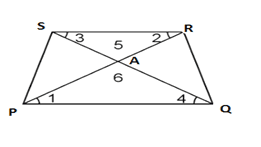Solution:

PQRS is a trapezium having PQ ǁ SR. The diagonals PR and QS intersect each other at a point A.

In ∆ PAQ and ∆ RAS, we have

1=2(Alternateangles) 3=4(Alternateangles) 5=6(Verticallyoppositeangle)

Therefore, ∆ PAQ  ~ ∆ RAS [By AAA similarity criterion]

Now, Area of (∆PAQ) / Area of (∆RAS)

= PQ2 / RS[If two triangles are similar then the ratio of their areas are equal to the square of the ratio of their corresponding sides]

= (2RS)/ RS[Therefore, PQ=RS]

Therefore, Area of (∆ PAQ)/ Area of (∆RAS)

= 4RS2/ RS = 4/1

Hence, the required ratio of the area of ∆PAQ and ∆RAS = 4:1

Question 3:

From the given figure, PQR and SQR are two triangles on the same base QR. If PS intersects QR at A, show that the area of triangle PQR / Area of triangle SQR = PA. SA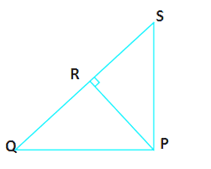Solution: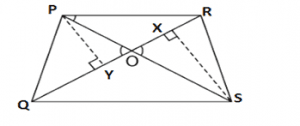Given,

PQR and SQR are the triangles in which have the same base QR. PS intersects QR at A.

To prove: Area of triangle PQR/ Area of triangle SQR = PA/ SA

Construction: Let us draw two perpendiculars PY and SX on line QR.

Proof:

We know that the area of a triangle = ½ x base x Height

Therefore, areaΔPQRareaΔSQR = 12QR×PY12QR×SX

In ∆ PYA and ∆ SXA,

PYA=SXA(Eachequalsto90) PAY=SAX(Verticallyoppositeangles) ΔPYAΔSXA(ByAAsimilaritycriterionPYSX) = PASA

=> area of triangle PQR/ area of triangle SQR

=> PA/SA

Question 4:

If the areas of two triangles are similar and equal then, prove that they are congruent.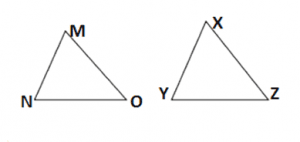Solution:

Given:

∆ MNO and ∆ XYZ are similar and equal in area.

To prove that: ∆ MNO  ∆ XYZ

Proof: Since, ∆ MNO ~ ∆ XYZ

Therefore, Area of (∆ MNO) / Area of (∆ XYZ) = NO2/ YZ2

=> NO2 / YZ2 = 1 [Since the area of triangle MNO = area of triangle XYZ]

=> NO/ YZ2

=> NO / YZ

Similarly, we can prove that

MN = XY and MO = XZ

Thus, ∆ MNO  ∆XYZ [ By SSS criterion of congruence]

Question 5:

X , Y and Z are respectively the mid-points of sides PQ, QR and RP of ΔPQR. Find the ratio of the areas of ΔXYZ and ΔPQR.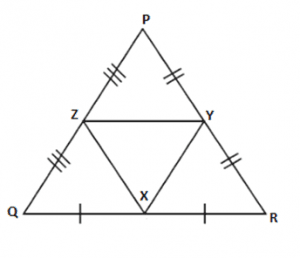Solution:

Given:

X, Y and Z are the mid-points of sides PQ, QR and RA respectively from the ΔPQR.

To Find: area(ΔXYZ) and area(ΔPQR)

Solution: In Δ PQR, we have
Z is the midpoint of PQ (Given)
Y is the midpoint of PR (Given)
So, by the mid-point theorem, we have
ZY || RQ and ZY = ½ RQ
⇒ ZY || RQ and ZY || QX [QX = ½ QR] ∴ QXYZ is parallelogram [Opposite sides of parallelogram are equal and parallel] Similarly, in Δ ZQX and Δ XYZ, we have
ZQ = XY (Opposite sides of parallelogram QXYZ)
ZX = ZX (Common)
QX = ZY (Opposite sides of parallelogram QXYZ)
∴ Δ ZQX ≅ ΔXYZ
Similarly, we can prove that
Δ PZY ≅ Δ XYZ
Δ YXR ≅ Δ XYZ
If triangles are congruent, then they are equal in area.
So, area(Δ FBD) = area(Δ DEF) …(i)
area(Δ AFE) = area(Δ DEF) …(ii)
and, area(Δ EDC) = area(Δ DEF) …(iii)
Now, area(Δ ABC) = area(Δ FBD) + area(Δ DEF) + area(Δ AFE) + area(Δ EDC) …(iv)
area(Δ ABC) = area(Δ DEF) + area(Δ DEF) + area(Δ DEF) + area(Δ DEF)
⇒ area(Δ DEF) = 1/4area(Δ ABC) [From (i), (ii) and (iii)] ⇒ area(Δ DEF)/area(Δ ABC) = 1/4
Hence, area(Δ DEF):area(Δ ABC) = 1:4

Question 6:

Prove that the ratio of the areas of two similar triangles is equal to the square of the ratio of their corresponding medians.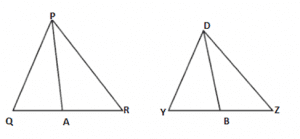Solution:

Given:

PA and XB are the medians of triangles PQR and XYZ respectively and Δ PQR ~ Δ XYZ

To prove: Area (Δ PQR)/ Area (Δ XYZ) = PA2 / XB2

Proof: Δ PQR ~ Δ XYZ (Given)

Therefore, Area (Δ PQR) / area (Δ XYZ ) = ( PQ2 / XY2) – – – – – – (1)

And, PQ / XY = QR / YZ = RP / ZX – – – – – (2)

PQXY=12QR12YZ=RXZX

In Δ PQA and Δ XYB, we have

Therefore, Q=Y(SinceΔPQRΔXYZ)

PQ / XY = QA / YB [Prove in (1)]

Therefore, Δ PQR ~ Δ XYZ [By SAS similarity criterion]

=> PQ/XY = PA/ XB – – – – – – – (3)

Therefore, Δ PQA ~ Δ XYB

The areas of two similar triangles are proportional to the squares of the corresponding sides.

Therefore, Area of triangle PQR / Area of triangle XYZ = PQ2 / XY2 = PA2/ XB2

Question 7:

Prove that the area of an equilateral triangle described on one side of a square is equal to half the area of the equilateral triangle described on one of its diagonals.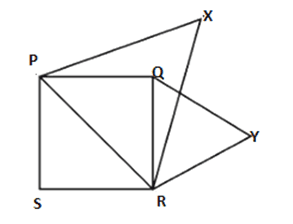Solution:

Given;

PQRS is a square whose one diagonal is PR. Δ PXR and Δ QYR are two equilateral triangles described on the diagonals PR and side QR of the square PQRS.

To prove:

Area of Δ QYR = ½ Area of Δ PXR

Proof:

Δ PXR and Δ QYR are both equilateral triangles (Given)

Therefore, Δ PXQ ~ Δ QYR [AAA similarity criterion]

Therefore, Area of Δ PXR / Area of Δ QYR = PR2 / QR2

(2QRQR)2=2QR2QR2=2[Since,Diagonal=2–√side=2–√QR]

=> Area (Δ PXR) = 2 x area (Δ QYR)

=> area (Δ QYR) = ½ area (ΔPXR)

Question 8:

Tick the correct solutions and explain.

PQR and QST are two equilateral triangles such that S is the midpoint of QR. The ratio of the areas of triangles PQR and QST is:

(i) 2 : 1

(ii) 1 : 2

(iii) 4 : 1

(iv) 1 : 4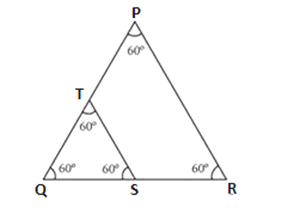Solution:

Δ PQR and Δ QST are two equilateral triangle. S is the midpoint of QR.

Therefore, QS = SR = ½ QR

Let each side of triangle be 2a

As, Δ PQR ~ Δ QST

Therefore, area (Δ PQR ) / area (Δ QST) = PQ2 / QS2 = (2a)/ (a) = 4a/ a = 4/1 = 4:1

Hence, The correct option is (iii)

Question 9:

Sides of two similar triangles are in the ratio 4 : 9. Areas of these triangles are in the ratio

(i) 2 : 3

(ii) 4: 9

(iii) 81 : 16

(iv) 16 : 81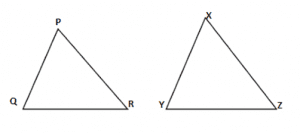Solution:

PQR and XYZ are two similarity triangles Δ PQR ~ Δ XYZ (Given)

And, PQ / XY = PR / XZ = QR / YZ = 4/9 (Given)

Therefore, Area of ΔPQR / Area of Δ XYZ = PQ2 / XY [the ratio of the areas of these triangles will be equal to the squares of the ratio of the corresponding sides]

Therefore, Area of Δ PQR / Area of Δ XYZ = (4/9)2 = 16/81

=> 16:81

Hence, the correct option is (iv)

### NCERT Solutions For Class 10 Maths Chapter 6 – Triangles – Exercise 6.5

Question 1:

Sides of the triangles are as follows:

1. i) 7 cm, 25 cm, 24 cm
2. ii) 3 cm, 6 cm, 8 cm

iii)           50 cm, 100 cm, 80 cm

1. iv) 5 cm, 12 cm, 13 cm

Determine which of them are right-angled triangles. Write the length of its hypotenuse.

Solution:

1. i) Sides of the triangle given are 7 cm, 25 cm and 24 cm.

Squaring the length of these sides we get 49 cm, 625 cm and 576 cm.

However, 49 + 576 = 625

(7)2 + (24)2 = (25)2

It satisfies the Pythagoras theorem. Hence, it is a right-angled triangle.

Length of Hypotenuse = 25 cm.

1. ii) Sides of the triangle given are 3 cm, 6 cm and 8 cm.

Squaring the length of these sides we get 9 cm, 36 cm and 64 cm.

However, 9 + 36 ≠ 64

(7)2 + (24)2 ≠ (25)2

It does not satisfy the Pythagoras theorem. Hence, it is not a right-angled triangle.

iii)           Sides of the triangle given are 50 cm, 100 cm and 80 cm.

Squaring the length of these sides we get 2500 cm, 10000 cm and 6400 cm.

However, 2500 + 6400 ≠ 10000

(50)2 + (80)2 ≠ (100)2

It does not the Pythagoras theorem. Hence, it is not a right-angled triangle.

1. iv) Sides of the triangle given are 5 cm, 12 cm and 13 cm.

Squaring the length of these sides we get 25 cm, 144 cm and 169 cm.

However, 25 + 144 = 169

(5)2 + (12)2 = (13)2

It satisfies the Pythagoras theorem. Hence, it is a right-angled triangle.

Length of Hypotenuse = 13 cm.

Question 2:

ABC is a right-angled triangle at point A. P is a point on BC such that AP ⊥ BC. Prove that AP2 = BP x PC.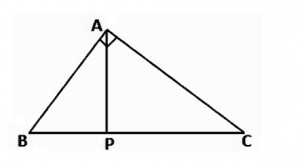Solution:

Given: ΔABC is a right-angled triangle at point A. P is appointed on BC such that AP ⊥ BC.

To prove: AP2 = BP x PC

Proof:

In ΔABC, we have

AB2 = AP+ BP2 [By Pythagoras theorem]

Or, AP= AB2 – BP2 ……….. (i)

In ΔAPC, we have

AC2 = AP+ PC2 [By Pythagoras theorem]

Or, AP= AC2 – PC2 ……….. (ii)

Adding (i) and (ii), we get

2AP2 = (AB2 – BP2) – (AC2 – PC2)

= BC2 – BP2 – PC2   [ BC2 = AB+ AC2]

= (BP + PC)2 – BP2 – PC2

= 2BP x PC

∴ AP= BP x PC

Question 3:

In the given figure, PQM is a right-angled triangle at P. Also PR ⊥ QM.

Show that

i) PQ2 = QR x QM

ii) PR= QR x MR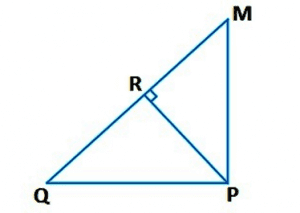Solution:

i) In ΔPMQ and ΔRPQ, we have

∠MPQ = ∠PRQ = 90o

∠PQM = ∠RQP (Common angle)

∴ΔPMQ ~ ΔRPQ [AA similarity criterion]

⇒ PQ/RQ = QM/PQ

⇒ PQ2 = QR x QM

ii) Let ∠RPQ = x

In ΔRQP,

∠RQP = 180o – 90o – x

∠RQP = 90o – x

Similarly, in ΔRPM

∠RPM = 90o – ∠RQP

= 90o – x

∠RMP = 180o – 90o – (90o – x)

∠RMP = x

In ΔRQP and ΔRPM, we have

∠RQP = ∠RPM

∠RPQ = ∠RMP

∠PRQ = ∠MRP = 90o

∴ ΔRQP ~ ΔRPM [By AAA similarity criterion]

⇒PR/MR = QR/PR

⇒PR2 = QR x MR

Question 4:

PQR is an isosceles right-angled triangle at point R. Prove that PQ2 = 2PR2.

Solution: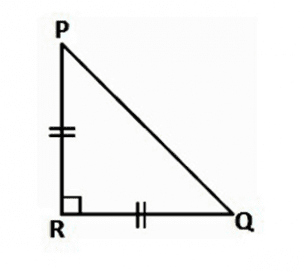Given: ΔPQR is an isosceles triangle right angled at R.

In ΔPRQ, ∠R = 90o

PR = QR (Given)

PQ2 = PR2 +QR2 [By Pythagoras theorem]

= PR+ PR[Since, PR = QR]

PQ2 = 2PR2

Question 5:

PQR is an isosceles triangle with PR = QR. Given that PQ2 = 2PR2. Prove that PQR is a right-angled triangle.

Solution: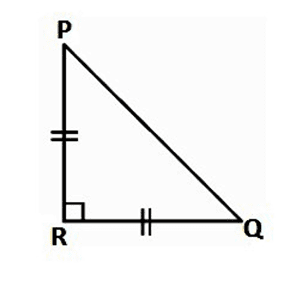Given that ΔPQR is an isosceles triangle having PR = QR and PQ2 = 2PR2

In ΔPRQ,

PR = QR (Given)

PQ2 = 2PR(Given)

= PR+ PR2

= PR+ QR[Since, PR = QR]

Hence, by Pythagoras theorem ΔPQR is a right-angle triangle.

Question 6:

PQR is an equilateral triangle of side 2a. Find each of its altitudes.

Solution: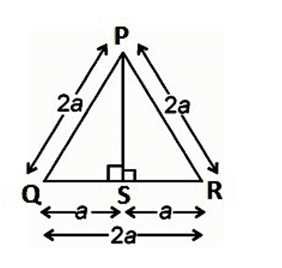Given: PQR is an equilateral triangle of side 2a.

Draw PS ⊥ QR

In ΔPSQ and ΔPSR, we have

PQ = PR [Given]

PS = PS [Given]

∠PSQ = ∠PSR = 90o

Therefore, ΔPSQ ≅ ΔPSR by RHS congruence.

In right-angled ΔPSQ,

(PQ)2 = (PS)2 + (QD)2

(2a)2 = (PS)2 + a2

⇒ (PS)2 = 4a2 – a2

⇒ (PS)2 = 3a2

⇒ PS = 3–√a

Question 7:

Sum of the squares of the sides of a rhombus is equal to the sum of the squares of its diagonals. Prove it.

Solution: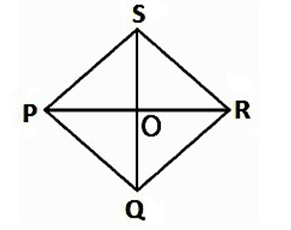Given: PQRS is a rhombus whose diagonals are PR and QS. They intersect at O.

To prove: PQ2 + QR2 + RS2+ PS2 = PR2 + QS2

Since the diagonals of as rhombus bisect each other ar tight angles.

Therefore, PO = RO and QO = SO

In ΔPOQ,

∠POQ = 90o

PQ2 = PO2 + QO2 ……. (i) [By Pythagoras]

Similarly,

PS= PO2 + SO2 ……. (ii)

RS2 = SO2 + RO2 ……. (iii)

QR2 = RO2 + QO2 ……. (iv)

Adding equation (i) + (ii) + (iii) + (iv) we get,

PQ2 + PS2 + RS+ QR= 2(PO2 + QO2+ RO2 + SO2)

= 4PO2 + 4QO2 [Since, PO = RO and QO = SO]

= (2PO)2 + (2QO2) = PR2 + QS2

Question 8:

In the given figure, O is a point in the interior of a triangle PQR.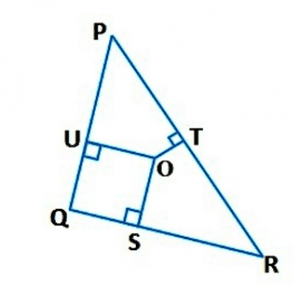OS ⊥ QR, OT ⊥ PR and OU ⊥ PQ. Show that

i) OP2 + OQ2 + OR2 – OS2 – OT2 – OU2 = PU2 + QS2 + RT2

ii) PU2 + QS2 + RT2 = PT2 + RS2 + QU2

Solution:

Join OP, OQ and OR

i) Applying Pythagoras theorem in ΔPOU, we have

OP2 = OU2 + PU2

Similarly, in ΔQOS

OQ2 = OS2 + QS2

Similarly, in ΔROT

OR2 = OT2 + TR2

OP2 + OQ2 + OR2 = OU2 + PU2 + OS2 + QS2 + OT2 + RT2

OP2 + OQ2 + OR2 – OS2 – OT2 – OU2 = PU2 + QS2 + RT2.

ii) PU2 + QS2 + RT2 = (OP2 – OT2) + (OR2 – OS2) + (OQ2 – OU2)

PU2 + QS2 + RT2 = PT2 + RS2 + QU2.

Question 9:

A ladder of 10 m length reaches a window of 8m above the ground. Find the distance of the foot of the ladder from the base of the wall.

Solution:Let QP be the wall and PR be the ladder,

Therefore, by Pythagoras theorem, we have

PR2 = PQ2 + QR2

102 = 82 + QR2

QR= 100 -64

QR2 = 36

QR = 6 m

Therefore, the distance of the foot of the ladder from the base of the wall is 6 m.

Question 10:

An airplane leaves an airport and flies due north at a speed of 1,000 km/hr. At the same time, another airplane leaves the same airport and flies due west at a speed of 1,200 km/hr. How far apart will be the two planes after one and half hours?

Solution: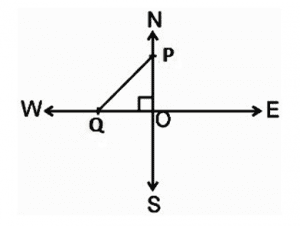Speed of the first aeroplane = 1000 km/he

Distance covered by first aeroplane due north in one and half hours

(OA) = 1000 x 3/2 km = 1500 km

Sped of the second aeroplane = 1200 km/hr

Distance covered by second aeroplane due west in one and half hours

(OB) = 1200 x 3/2 km = 1800 km

In right angle ΔPOQ, we have

PQ2 = PO2 + OQ2

⇒ PQ2 = (1500)2 + (1800)2

⇒ PQ = 2250000+3240000−−−−−−−−−−−−−−−√

5490000−−−−−−−√

30061−−√ km

Hence, the distance between two aeroplanes will be 30061−−√ km.

Question 11:

Two planes of heights 6 m and 11m respectively stand on a plane ground. If the distance between the feet of the poles is 12 m, find the distance between their tops.

Solution: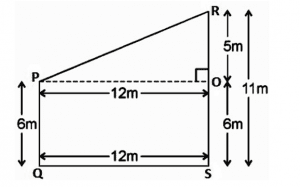Let PQ and RS be the poles of height 6 m and 11 m respectively.

Therefore RO = 11 – 6 = 5 m

From the figure, it can be observed that PO = 12 m

Applying Pythagoras theorem for ΔPOR, we get

PO2 = OR2 + PR2

(12m)+ (5m)2 = (AC)2

AC2 = (144+25) m = 169 m

AC = 13 m

Therefore, the distance between their tops is 13 m.

Question 12:

S and T are points on the sides RP and RQ respectively of a triangle PQR right angled at R. Prove that PT2 + QS2= PQ2 + ST2

Solution: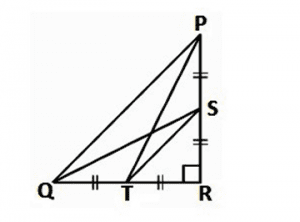Applying Pythagoras theorem in ΔPRT, we get

PR2 + RT2 = PT2 ………. (i)

Applying Pythagoras theorem in ΔQRS, we get

QR2 + RS2 = QS2 ………. (ii)

Adding (i) + (ii), we get

PR2 + RT+ QR2 + RS= PT+ QS2 ………. (iii)

Applying Pythagoras theorem in ΔRST, we get

ST2 = RS2 + RT2

Applying Pythagoras theorem in ΔPQR, we get

PQ2 = PR2 + RQ2

Putting these values in equation (iii), we get

ST2 + PQ2 = PT2 + QS2.

Question 13:

The perpendicular from P on side QR of a ΔPQR intersects QR at S such that SQ = 3RS. Prove that 2PQ2 = 2PR2 + QR2.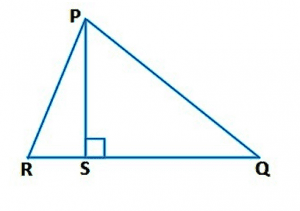Solution:

Given that in ΔPQR, we have

PS ⊥ QR and SQ = 3RS

In right-angled triangles PSQ and PSR, we have

PQ2 = PS2 + QS2 ……. (i)

PR2 = PS2 + SR2 ……. (ii) [By Pythagoras theorem]

(ii) – (i), we get

PQ– PR= QS2 – SR2

= 9SR2 – SR2 [Since, SQ = 3SR]

= 8(QR/4)2 [Since, QR = SQ + RS = 3RS +RS = 4RS]

Therefore, PQ2 – PR2 = QR2/2

⇒ 2(PQ2 – PR2) = QR2

Therefore, 2PQ2 = 2PR2 + QR2.

Question 14:

In an equilateral triangle PQR, S is a point on side QR such that QS = 1/3 QR. Prove that 9PS2 = 7PQ2.

Solution: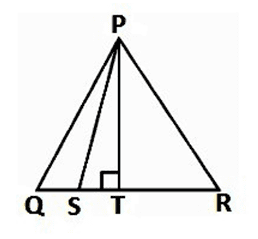Let the side of the equilateral triangle be a, and PT be the altitude of ΔPQR.

∴ QT = TR = QR/2 = a/2

And, PT = 3a2

Given that, QS = 1/3 QR

∴ QS = a/3

ST = QT – QS = a/2 – a/3 = a/6

Applying Pythagoras theorem in ΔPST, we get

PS2 = PT2 + ST2

PS2 = (a32)2 + (a6)2

3a24 + (a236)

28a236

79 AB2

⇒ 9AD2 = 7AB2

Question 15:

In an equilateral triangle, prove that three times the square of one side is equal to four times the square of its altitudes.

Solution: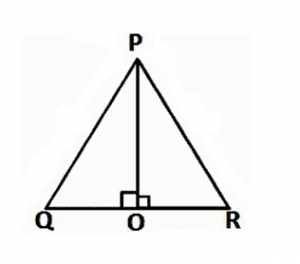Let the side of the equilateral triangle be a, and PO be the altitude of ΔPQR.

∴ QO = OR = QR/2 = a/2

Applying Pythagoras theorem in ΔPQO, we get

PQ2 = PO2 + QO2

a2=AE2+(a2)2 AE2=a2a24 AE2=3a24

4AE2 = 3a2

⇒ 4 x (Square of altitude) = 3 x (Square of one side)

Question 16:

Choose the correct solution and justify:

In ΔPQR, PQ = 6√3 cm, PR = 12 cm and QR = 6 cm.

The angle Q is:

i) 120o

ii) 60o

iii) 90o

iv) 45o

Solution: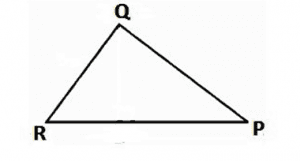Given that, PQ = 6√3 cm, PR = 12 cm and QR = 6 cm

We can observe that

PQ2 = 108

PR2 = 144

And, BC2 = 36

PQ2 + BC2 = PR2

The given triangle, ΔPQR is satisfying Pythagoras theorem.

Therefore, the triangle is a right-angled triangle at B.

∴ ∠B = 90o

Hence, the correct option is (iii)

From the above problems and solutions, you can get a list of a variety of questions covering all the topics in NCERT Solutions for Maths Class 10 chapter 6. This solutions book could work as worksheets for students to prepare for the CBSE Class 10 Board exams.